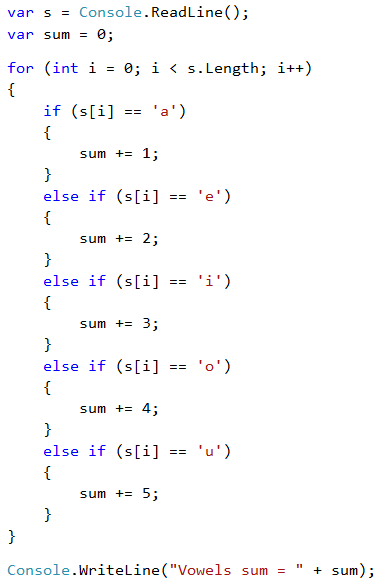# Problem: Sum of Vowels

Write a program that inputs text (string), calculates and prints the sum of the values of vowels according to the table below:

a e i o u
1 2 3 4 5

## Sample Input and Output

Input Output Input Output
hello 6
(e+o = 2+4 = 6)
bamboo 9
(a+o+o = 1+4+4 = 9)
hi 3
(i = 3)
beer 4
(e+e = 2+2 = 4)

## Video: Sum of Vowels

Watch this video lesson to learn how to sum the vowels of given text: https://youtu.be/9hi9G3vRA0U.

## Hints and Guidelines

We read the input text s, null the sum and run a loop from 0 to s.Length-1 (text length -1). We check each letter s[i] and verify if it is a vowel, and accordingly, add its value to the sum.## Testing in the Judge System

Test your solution here: https://judge.softuni.org/Contests/Practice/Index/510#8.